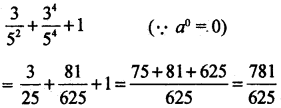# 1st PUC Basic Maths Question Bank Chapter 3 Theory of Indices

Students can Download Basic Maths Chapter 3 Theory of Indices Questions and Answers, Notes Pdf, 1st PUC Basic Maths Question Bank with Answers helps you to revise the complete Karnataka State Board Syllabus and score more marks in your examinations.

## Karnataka 1st PUC Basic Maths Question Bank Chapter 3 Theory of Indices

Question 1.
3(5-2) + $$\left(\frac{5}{3}\right)^{-4}$$ + (a + 5)0Question 2.
Show that $$\left(\frac{x^{b}}{x^{c}}\right)^{a}-\left(\frac{x^{c}}{x^{a}}\right)^{b} \cdot\left(\frac{x^{a}}{x^{b}}\right)^{c}=1$$
LHS = (xb-c)a (xc-a)3 (xa-b)c
= xab-bc .xbc-ab .xac-bc
= xab-bc+bc+-ab+ac-bc
= x0
= 1
= RHSQuestion 3.
If pqr = 1 Show that $$\frac{1}{1+p+q^{-1}}+\frac{1}{1+q+r^{-1}}+\frac{1}{1+r+p^{-1}}=1$$Question 4.
If ax = by = and b2 = ac. Show that $$\frac{1}{x}+\frac{1}{z}=\frac{2}{y}$$
Let ax = by = cz = k (say)
⇒ ax = k, by = k, Cz = k
⇒ a = k1/x; b = k1/y; c = k1/z
By data b2 = ac
(k1/y)2 = k1/x .k1/z
k2/y = k1/x+1/z
⇒ $$\frac{2}{y}=\frac{1}{x}+\frac{1}{z}$$ (∵bases are same)Question 5.
If 651/x = 131/y = 51/z show that x=y+z.